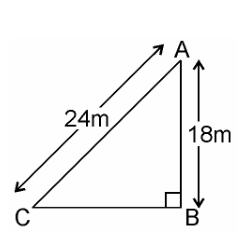# A guy wire attached to a vertical pole of height 18 m is 24 m long and has a stake attached to the other end. How far from the base of the pole should the stake be driven so that the wire will be taut?

Given

A guy wire attached to a vertical pole of height 18 m is 24 m long and has a stake attached to the other end.

We need to find the distance of the foot of the ladder from the base of the wallAB is a pole = 18m

AC is a wire = 24m

BC = ?

In a right angled triangle ABC,

By Pythagoras theorem

(AC)2 = (AB)2 + (BC)2

(24)2 = (18)2 + (BC)2

576 = 324 + (BC)2

(BC)2 = 576 – 324

We get,

(BC)2 = 252

BC = √252

BC = √(2 x 2 x 3 x 3 x 7)

BC = 2 x 3√7

We get,

BC = 6√7

Therefore, the stake may be placed at distance of 6√7 from the base of the pole.(0)(0)